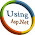# Introduction: Hello dears, in this article i will explain that how we can createVisualization: Gauge Chartusing asp.net. This is very helpful artucle for the asp.net deveploer to creating a mast graph in asp.net. This type of chart you can create to showing  the percentage of the student,Cricket scoreetc

Implementation: Here i am taking the reference from the below link . http://code.google.com/apis/chart/interactive/docs/gallery/gauge.html
Google use javascript code for the implementation of this graph. I am using same javascript code but am merging also my asp.net experience with this code. And create the same graph in Asp.Net.
Code for .aspx page:
<%--the below two javascripts  are needed to run this program you can
<body>
<form id="form1" runat="server">
<div>
<%--here i am placing Literal control from the toolbox
to display a grapg  --%>
<asp:Literal ID="lt" runat="server"></asp:Literal>
</div>
<div id='chart_div'></div>
</form>
</body>
</html>
Code for aspx.cs page:

using System.Data;
using System.Linq;
using System.Web;
using System.Web.Security;
using System.Web.UI;
using System.Web.UI.HtmlControls;
using System.Web.UI.WebControls;
using System.Web.UI.WebControls.WebParts;
using System.Xml.Linq;
using System.Text;
using System.Data.SqlClient;
using System.IO;
using System.Data.Sql;

public partial class speedometer_gauge : System.Web.UI.Page
{
// here i am taking a object of StringBuilder class named str
StringBuilder str = new StringBuilder();
SqlConnection con = new SqlConnection();
protected void Page_Load(object sender, EventArgs e)
{
// here i am declare connection
con.ConnectionString = ConfigurationManager.ConnectionStrings["cn"].ConnectionString;
con.Open();
if (Page.IsPostBack == false)
{
// here i am calling function  chart_bind(); in the page load event of the page
bind_chart();
}

}
private void bind_chart()
{
// here i am using SqlDataAdapter for the sql server select query
// here am taking datatable
DataTable dt = new DataTable();
try
{
// here datatale dt is fill wit the adp
// this string m catching in the stringbuilder class
// in the str m writing same javascript code that is given by the google.
// my data that will come from the sql server
// below code is same like as google's javascript code
str.Append(@" <script type='text/javascript'>
function drawChart() {
// inside the below line dt.Rows.Count explain that
// how many rows comes in dt or total rows

Int32 i;
for (i = 0; i <= dt.Rows.Count - 1; i++)
{
// i need this type of output "  data.setValue(0, 0, 'Memory'); so on  in the first line so for this
//m using i for the 0,1& 2 and so on . and after this i am writting zero and after this student_name using datatable
str.Append("data.setValue( " + i + "," + 0 + "," + "'" + dt.Rows[i]["student_name"].ToString() + "');");
// i need this type of output "   data.setValue(0, 1, 80);
//so on  in the first line so for this
//m using i for the 0,1& 2 and so on . and after this i am writting zero and after this percentage using datatable
str.Append("data.setValue(" + i + "," + 1 + "," + dt.Rows[i]["percentage"].ToString() + ") ;");

//   str.Append("['" + (dt.Rows[i]["student_name"].ToString()) + "'," + dt.Rows[i]["average_marks"].ToString() + "],");
}

// in the below line i am setting the height and width of the Gauge using your own requrirement
str.Append(" var options = {width: 800, height: 300, redFrom: 90, redTo: 100,");
//  str.Append(" var chart = new google.visualization.BarChart(document.getElementById('chart_div'));");
str.Append("yellowFrom:75, yellowTo: 90, minorTicks: 5};");
str.Append(" chart.draw(data, options);}");
str.Append("</script>");
lt.Text = str.ToString().TrimEnd(',');

con.Close();
}
catch
{

}
finally
{
con.Close();
}
}
}
See output in this image:

Sql Script for database:

/****** Object:  Table [dbo].[tb_student_percentage]    Script Date: 07/15/2011 16:55:32 ******/
IF  EXISTS (SELECT * FROM sys.objects WHERE object_id = OBJECT_ID(N'[dbo].[tb_student_percentage]') AND type in (N'U'))
DROP TABLE [dbo].[tb_student_percentage]
GO
/****** Object:  Table [dbo].[tb_student_percentage]    Script Date: 07/15/2011 16:55:32 ******/
SET ANSI_NULLS ON
GO
SET QUOTED_IDENTIFIER ON
GO
IF NOT EXISTS (SELECT * FROM sys.objects WHERE object_id = OBJECT_ID(N'[dbo].[tb_student_percentage]') AND type in (N'U'))
BEGIN
CREATE TABLE [dbo].[tb_student_percentage](
[id] [bigint] IDENTITY(1,1) NOT NULL,
[student_name] [varchar](50) COLLATE SQL_Latin1_General_CP1_CI_AS NULL,
[percentage] [varchar](50) COLLATE SQL_Latin1_General_CP1_CI_AS NULL,
CONSTRAINT [PK_tb_student_percentage] PRIMARY KEY CLUSTERED
(
[id] ASC
)WITH (PAD_INDEX  = OFF, STATISTICS_NORECOMPUTE  = OFF, IGNORE_DUP_KEY = OFF, ALLOW_ROW_LOCKS  = ON, ALLOW_PAGE_LOCKS  = ON)
)
END
GO
SET IDENTITY_INSERT [dbo].[tb_student_percentage] ON
INSERT [dbo].[tb_student_percentage] ([id], [student_name], [percentage]) VALUES (1, N'bharat', N'85')
INSERT [dbo].[tb_student_percentage] ([id], [student_name], [percentage]) VALUES (2, N'vimal', N'56')
INSERT [dbo].[tb_student_percentage] ([id], [student_name], [percentage]) VALUES (3, N'amar', N'58')
INSERT [dbo].[tb_student_percentage] ([id], [student_name], [percentage]) VALUES (4, N'pardeep', N'58')
INSERT [dbo].[tb_student_percentage] ([id], [student_name], [percentage]) VALUES (5, N'munish', N'99')
INSERT [dbo].[tb_student_percentage] ([id], [student_name], [percentage]) VALUES (6, N'rajjo', N'100')
SET IDENTITY_INSERT [dbo].[tb_student_percentage] OFF

Conclusion
Through this article, you have learned how to create Visualization: Gauge Chart using the google’s javascript code as well as asp.net code.

Okas  guys, enjoy this code. Meet in next article again.

Thanx

1.Hi,

Nice article. It helped me to generate the chart but can you please tell me how to save the generated image from the browser to either excel or pdf?
1.2.3.